NCERT Solutions for Class 7 Maths Chapter 11 Perimeter and Area Ex 11.1 are part of NCERT Solutions for Class 7 Maths. Here we have given NCERT Solutions for Class 7 Maths Chapter 11 Perimeter and Area Ex 11.1.

 Board CBSE Textbook NCERT Class Class 7 Subject Maths Chapter Chapter 11 Chapter Name Perimeter and Area Exercise Ex 11.1 Number of Questions Solved 8 Category NCERT Solutions

## NCERT Solutions for Class 7 Maths Chapter 11 Perimeter and Area Ex 11.1

Question 1.
The length and the breadth of a rectangular piece of land is 500 m and 300 m respectively. Find
(i) its area
(ii) the cost of the land, if 1 m2 of the land cost ₹ 10,000.
Solution:Here, length = 500 m, breadth = 300 m
(i) Area = length × breadth = (500 × 300) m2 = 1,50,000 m2
(ii) Cost of land at the rate of ₹ 10,000 per 1 m2 = ₹ (10,000 × 1,50,000) = ₹ 1,50,00,00,000.

Question 2.
Find the area of a square park whose perimeter is 320 m.
Solution:Question 3.
Find the breadth of a rectangular plot of land, if its area is 440 m2 and the length is 22 m. Also, find its perimeter.
Solution: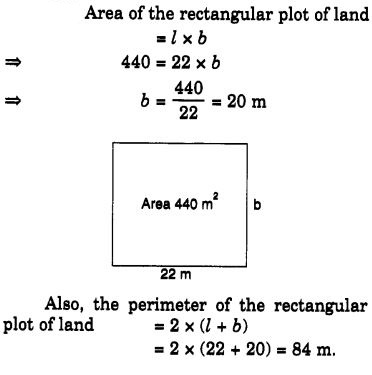Question 4.
The perimeter of a rectangular sheet is 100 cm. If the length is 35 cm, find its breadth. Also find the area.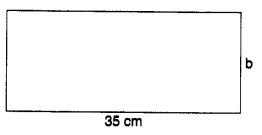Solution: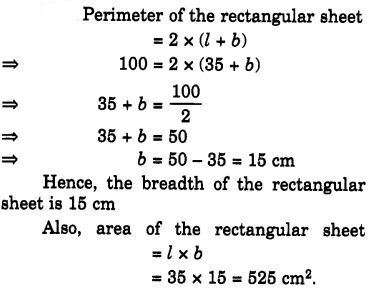Question 5.
The area of a square park is the same as a rectangular park. If the side of the square park is 60 m and the length of the rectangular park is 90 m, find the breadth of the rectangular park.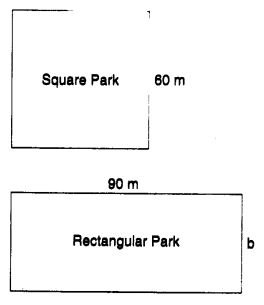Solution: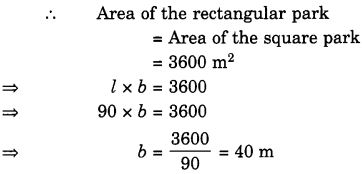Hence, the breadth of the rectangular park is 40 m.

Question 6.
A wire is in the shape of a rectangle. Its length is 40 cm and breadth is 22 cm. If the same wire is rebent in the shape of a square, what will be the measure of each side? Also, find which shape encloses more area?
Solution: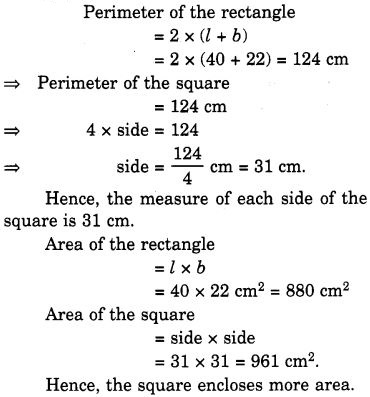Question 7.
The perimeter of a rectangle is 130 cm. If the breadth of the rectangle is 30 cm, find its length. Also, find the area of the rectangle.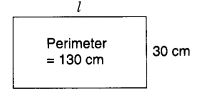Solution: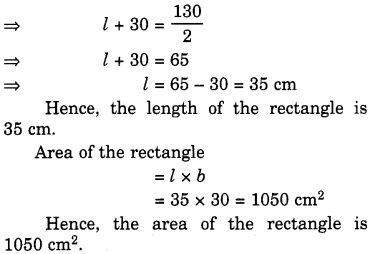Question 8.
A door of length 2 m and breadth 1 m is fitted in a wall. The length of the wall is 4.5 m and the breadth is 3.6 m (figure). Find the cost of whitewashing the wall, if the rate of whitewashing the wall is ₹ 20 per m2.
Solution:We hope the NCERT Solutions for Class 7 Maths Chapter 11 Perimeter and Area Ex 11.1 help you. If you have any query regarding NCERT Solutions for Class 7 Maths Chapter 11 Perimeter and Area Ex 11.1, drop a comment below and we will get back to you at the earliest.